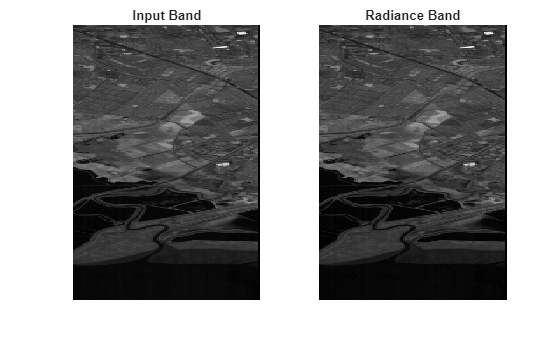Since R2020b

## Syntax

``newhcube = dn2radiance(hcube)``
``newhcube = dn2radiance(hcube,'BlockSize',blocksize)``

## Description

example

````newhcube = dn2radiance(hcube)` converts the pixel values of the hyperspectral data cube from digital number to radiance values. The function returns a new `hypercube` object and the pixel values of the data cube are the top of atmosphere (TOA) radiances.```
````newhcube = dn2radiance(hcube,'BlockSize',blocksize)` specifies the block size for block processing of the hyperspectral data cube by using the name-value pair argument `'BlockSize'`.The function divides the input image into distinct blocks, processes each block, and then concatenates the processed output of each block to form the output matrix. Hyperspectral images are multi-dimensional data sets that can be too large to fit in system memory in their entirety. This can cause the system to run out of memory while running the `dn2radiance` function. If you encounter such an issue, perform block processing by using this syntax.For example, `dn2radiance(hcube,'BlockSize',[50 50])` divides the input image into non-overlapping blocks of size 50-by-50 and then computes the radiance values for pixels in each block. NoteTo perform block processing by specifying the `'BlockSize'` name-value pair argument, you must have MATLAB® R2021a or a later release. NoteThis function requires the Image Processing Toolbox™ Hyperspectral Imaging Library. You can install the Image Processing Toolbox Hyperspectral Imaging Library from Add-On Explorer. For more information about installing add-ons, see Get and Manage Add-Ons.The Image Processing Toolbox Hyperspectral Imaging Library requires desktop MATLAB, as MATLAB Online™ or MATLAB Mobile™ do not support the library. ```

## Examples

collapse all

Read hyperspectral data into the workspace.

`hcube = hypercube('EO1H0440342002212110PY_cropped.hdr');`

Determine the bad spectral band numbers using the `BadBands` parameter in the metadata.

`bandNumber = find(~hcube.Metadata.BadBands);`

Remove the bad spectral bands from the data cube.

`hcube = removeBands(hcube,'BandNumber',bandNumber);`

Compute the radiance values using the `dn2radiance` function.

`newhcube = dn2radiance(hcube);`

Read and display a spectral band image in the input and the output radiance data.

```inputBand = hcube.DataCube; radianceBand = newhcube.DataCube; band = 80; figure subplot(1,2,1) imagesc(inputBand(:,:,band)) title('Input Band') axis off subplot(1,2,2) imagesc(radianceBand(:,:,band)) title('Radiance Band') axis off colormap gray ```## Input Arguments

collapse all

Input hyperspectral data, specified as a `hypercube` object. The `DataCube` property of the `hypercube` object stores the hyperspectral data cube. To convert the pixel values in digital numbers to radiance values, the `Metadata` property of the `hypercube` object must contain the `Gain` and `Offset` fields.

Size of the data blocks, specified as a 2-element vector of positive integers. The elements of the vector correspond to the number of rows and columns in each block, respectively. The size of the data blocks must be less than the size of the input image. Dividing the hyperspectral images into smaller blocks enables you process large data sets without running out of memory.

• If the `blocksize` value is too small, the memory usage of the function reduces at the cost of increased execution time.

• If the `blocksize` value is large or equal to the input image size, the execution time reduces at the cost of increased memory usage.

Example: `'BlockSize',[20 20]` specifies the size of each data block as 20-by-20.

## Output Arguments

collapse all

Output hyperspectral data, returned as a `hypercube` object. The pixels values of the output data cube are radiances specifying the amount of radiation from the surface being imaged. The radiance values are computed from digital numbers by using the equation:

Gain and Bias are the gain and offset values for each spectral bands respectively. The `Metadata` property of `hypercube` object contains the gain and the offset values.

## Version History

Introduced in R2020b